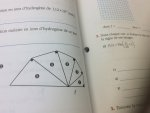Formula for length of the hypothenuse of a selected triangle in this problem

Thepotatom

New member
Hi, I have this homework which includes the picture I attached.. The figure is made in a way the the hypothenuse of the previous triangle is the long side of the next one. The text (it’s in french), says that the area of any of those right triangle ‘‘n’’ is: A(n)= 6(5/4)^(2n-2). It also said that the side lenghts of the first right triangle were 3,4 and 5. The question only asked to find the area of a 7th triangle, which wasn’t too hard, but I wondered if it was possible to come up with a formula for the hypothenuse of any triangle ‘‘n’’.
I tried without much success or progress but I was just curious.
Thanks,
Thomas

Jomo

Elite Member
Hi, I have this homework which includes the picture I attached.View attachment 11020. The figure is made in a way the the hypothenuse of the previous triangle is the long side of the next one. The text (it’s in french), says that the area of any of those right triangle ‘‘n’’ is: A(n)= 6(5/4)^(2n-2). It also said that the side lenghts of the first right triangle were 3,4 and 5. The question only asked to find the area of a 7th triangle, which wasn’t too hard, but I wondered if it was possible to come up with a formula for the hypothenuse of any triangle ‘‘n’’.
I tried without much success or progress but I was just curious.
Thanks,
Thomas
Can we see your work? Also, can you please define n. You can use any variable you like but you must define it! It would be nice to see what you got for the hypotenuse for the 2nd, 3rd, 4th and 5th triangle as this is one way for you to to see if you are getting a pattern.

Thepotatom

New member
Sure. N is defined as the number of a triangle, the first triangle being n=1, the second triangle being n=2, etc. And I found that the triangles are all similar, and because we know the side lengths of one triangle (3,4,5) we know the ratio of either side on another side. For example, the ratio of the shorter leg on the longer leg is 3/4. That’s where I am now.

tkhunny

Moderator
Staff member
Sure. N is defined as the number of a triangle, the first triangle being n=1, the second triangle being n=2, etc. And I found that the triangles are all similar, and because we know the side lengths of one triangle (3,4,5) we know the ratio of either side on another side. For example, the ratio of the shorter leg on the longer leg is 3/4. That’s where I am now.
We have 1) 3, 4, 5 ==> 2) ??,5,??

Dr.Peterson

Elite Member
Hi, I have this homework which includes the picture I attached.View attachment 11020. The figure is made in a way the the hypothenuse of the previous triangle is the long side of the next one. The text (it’s in french), says that the area of any of those right triangle ‘‘n’’ is: A(n)= 6(5/4)^(2n-2). It also said that the side lenghts of the first right triangle were 3,4 and 5. The question only asked to find the area of a 7th triangle, which wasn’t too hard, but I wondered if it was possible to come up with a formula for the hypothenuse of any triangle ‘‘n’’.
I tried without much success or progress but I was just curious.
Thanks,
Thomas
I don't see that you have said how the other leg of each triangle is determined. Does it say that they are all similar, or that the angles are the same, or something like that?

And are you saying that A(n)= 6(5/4)^(2n-2) was part of the question, or the answer?

If you know the triangles are similar, then the hypotenuses form a geometric progression (as do the areas), and it's easy to answer your question.

Jomo

Elite Member
Sure. N is defined as the number of a triangle, the first triangle being n=1, the second triangle being n=2, etc. And I found that the triangles are all similar, and because we know the side lengths of one triangle (3,4,5) we know the ratio of either side on another side. For example, the ratio of the shorter leg on the longer leg is 3/4. That’s where I am now.
Thanks for defining n. I knew what it meant but when one communicates to other mathematically they MUST define their variables. Also note that N and n are not the same variable. So what is the area of triangle 2/ area of triangle 1. The area of triangle 3 / area of triangle 2? ... .We are not making progress because we have to ask you the same things multiple times. What is the area of t1, area of t2,.... Note that ti is the area of the ith triangle.

Last edited: